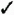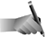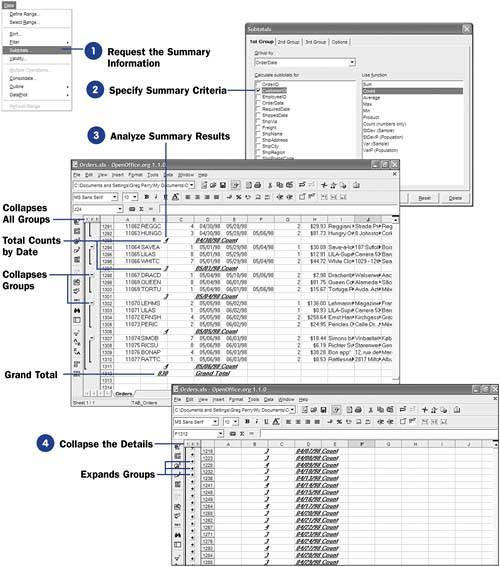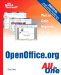# 79 Compute Table Totals and Subtotals

 <  Day Day Up  >

When working with any kind of financial database information, the ability to calculate subtotals and totals, based on sorted data, becomes necessary. For example, you might want to see all the total sales from a given region or ZIP Code. If any sales are down in one area, you can get your Marketing Department to step up their efforts in that area.

Before You Begin76 Import Data into a Calc Database77 Sort Calc Database Data78 Filter Data That You Want to See

Calc can summarize database data for you based on any of the following criteria:

• Sum ” The added total of data

• Count ” The number of items in the data range

• Average ” The calculated intermediate value in a range of data

• Min or max amounts ” The lowest or highest value in the data

• Product ” The multiplied result of the data values

• Standard deviation (of a sample or population) ” A statistic that measures how well dispersed values in a data range are

• Variance (of a sample or population) ” The square of the standard deviation used for statistical measurements

NOTEThe 2nd Group and 3rd Group tabs are for performing statistical analysis, which you may want to do when you have multiple divisions in multiple areas, or countries , and want to group first by area, then by division, and then by an individual field, such as sales per customer. The resulting summarized groups would show a subtotal of each customer, then each division, and finally each area, with a grand total at the bottom.

1. Request the Summary Information

Click anywhere within the data range that you want to summarize. Select Data, Subtotals to open the Subtotals dialog box.

2. Specify Summary Criteria

The Subtotals dialog box opens with the 1st Group tab displayed. This is where you specify the first grouping you want to see. Often, you'll select only one group, even though Calc supports up to four subtotal groups. Specify the Group by value (such as a date), followed by a selection of the field you want a subtotal for (such as CustomerID ). You then must tell Calc how the subtotals are to be grouped, such as by Count . In this case, you are requesting a count of each customer's order, grouped by date. If a customer orders three times on one day, that customer's daily orders should appear together when you click OK to display the summary.3. Analyze Summary Results

Calc produces a summarized version of your data range. At first, the summary may look confusing because Calc inserts counts (or sums or averages or other summary items, depending on your selection) throughout your data.

The count totals, for example, might show by each date in your data how many orders you had on that date. At the end of the report, Calc provides a grand total of all the counts.

4. Collapse the Details

You can click the minus sign to the left of a row number to collapse that group's detail. The minus sign then becomes a plus sign. By collapsing various types of detail in your summary (by clicking the 1, 2 , or 3 button to the left of the row number), you can get a count of the grand total only, of each group, of each group with all the details shown, respectively.

 <  Day Day Up  >Sams Teach Yourself OpenOffice.org All In One
ISBN: 0672326183
EAN: 2147483647
Year: 2003
Pages: 205
Authors: Greg Perry